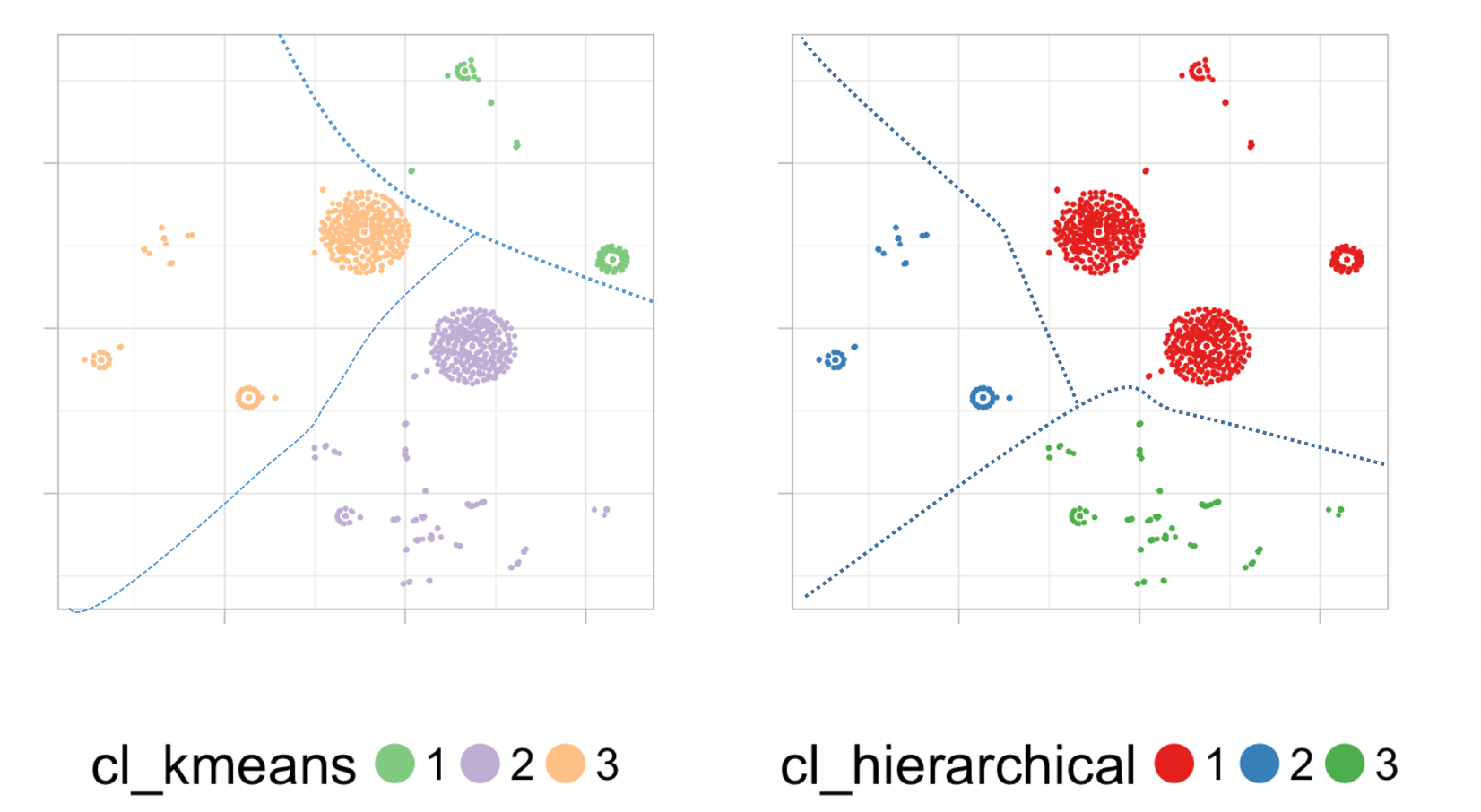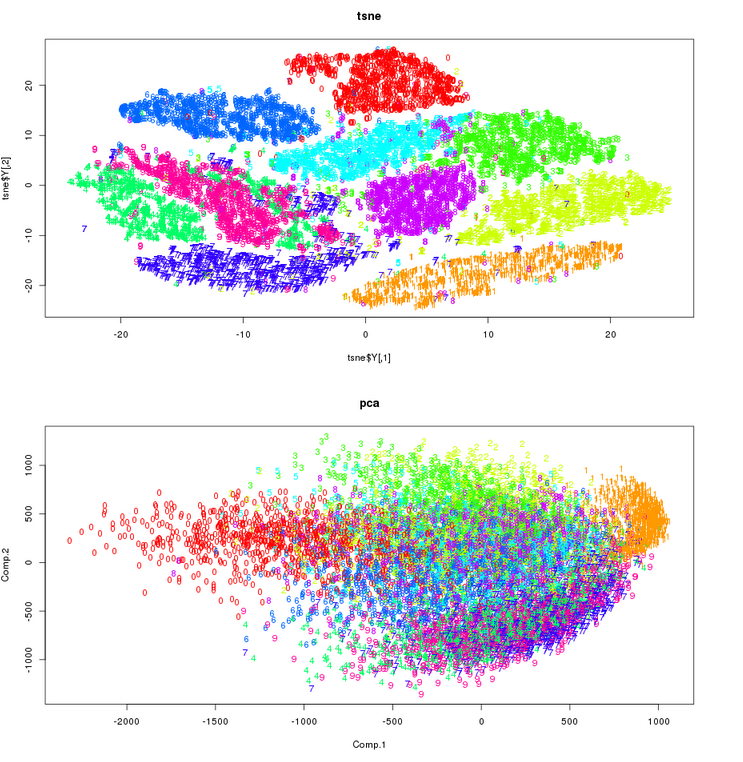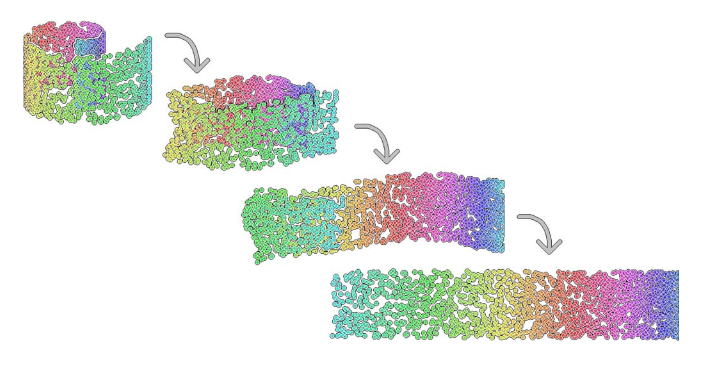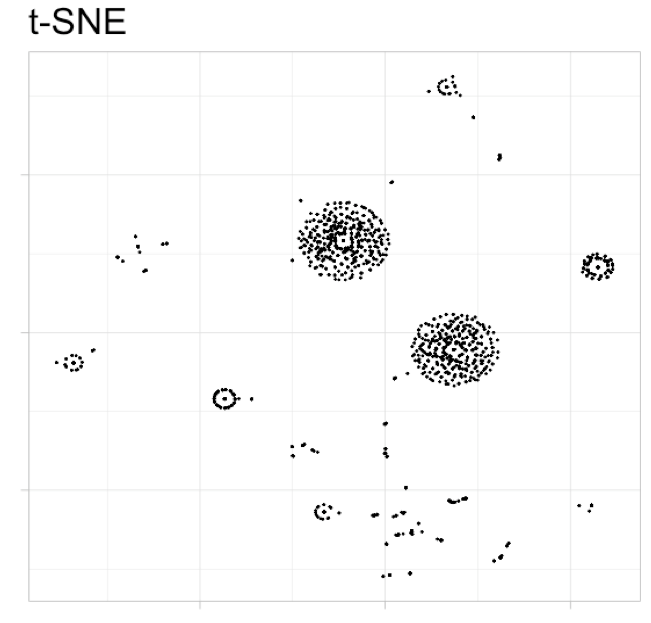[This article was first published on R - Data Science Heroes Blog, and kindly contributed to R-bloggers]. (You can report issue about the content on this page here)
Want to share your content on R-bloggers? click here if you have a blog, or here if you don't.

### Playing with dimensionsHi there! This post is an experiment combining the result of t-SNE with two well known clustering techniques: k-means and hierarchical. This will be the practical section, in R.But also, this post will explore the intersection point of concepts like dimension reduction, clustering analysis, data preparation, PCA, HDBSCAN, k-NN, SOM, deep learning…and Carl Sagan!

### PCA and t-SNE

For those who don’t know t-SNE technique (official site), it’s a projection technique -or dimension reduction- similar in some aspects to Principal Component Analysis (PCA), used to visualize N variables into 2 (for example).

When the t-SNE output is poor Laurens van der Maaten (t-SNE’s author) says:

As a sanity check, try running PCA on your data to reduce it to two dimensions. If this also gives bad results, then maybe there is not very much nice structure in your data in the first place. If PCA works well but t-SNE doesn’t, I am fairly sure you did something wrong.

In my experience, doing PCA with dozens of variables with:

• some extreme values
• skewed distributions
• several dummy variables,

Check out this example comparing the two methods:Makes sense, doesn’t it?

### Surfing higher dimensions ?

Since one of the t-SNE results is a matrix of two dimensions, where each dot reprents an input case, we can apply a clustering and then group the cases according to their distance in this 2-dimension map. Like a geography map does with mapping 3-dimension (our world), into two (paper).

t-SNE puts similar cases together, handling non-linearities of data very well. After using the algorithm on several data sets, I believe that in some cases it creates something like circular shapes like islands, where these cases are similar.

However I didn’t see this effect on the live demonstration from the Google Brain team: How to Use t-SNE Effectively. Perhaps because of the nature of input data, 2 variables as input.

#### The swiss roll data

t-SNE according to its FAQ doesn’t work very well with the swiss roll -toy- data. However it’s a stunning example of how a 3-Dimension surface (or manifold) with a concrete spiral shape *is unfols like paper thanks to a reducing dimension technique.

The image is taken from this paper where they used the manifold sculpting technique.### Now the practice in R!

t-SNE helps make the cluster more accurate because it converts data into a 2-dimension space where dots are in a circular shape (which pleases to k-means and it’s one of its weak points when creating segments. More on this: K-means clustering is not a free lunch).

Sort of data preparation to apply the clustering models.

```library(caret)
library(Rtsne)

######################################################################
## The WHOLE post is in: https://github.com/pablo14/post_cluster_tsne
######################################################################

## Rtsne function may take some minutes to complete...
set.seed(9)
tsne_model_1 = Rtsne(as.matrix(data_tsne), check_duplicates=FALSE, pca=TRUE, perplexity=30, theta=0.5, dims=2)

## getting the two dimension matrix
d_tsne_1 = as.data.frame(tsne_model_1\$Y)
```

Different runs of `Rtsne` lead to different results. So more than likely you will not see exactly the same model as the one present here.

According to the official documentation, `perplexity` is related to the importance of neighbors:

• “It is comparable with the number of nearest neighbors k that is employed in many manifold learners.”
• “Typical values for the perplexity range between 5 and 50”

Object `tsne_model_1\$Y` contains the X-Y coordinates (`V1` and `V2` variables) for each input case.

Plotting the t-SNE result:

```## plotting the results without clustering
ggplot(d_tsne_1, aes(x=V1, y=V2)) +
geom_point(size=0.25) +
guides(colour=guide_legend(override.aes=list(size=6))) +
xlab("") + ylab("") +
ggtitle("t-SNE") +
theme_light(base_size=20) +
theme(axis.text.x=element_blank(),
axis.text.y=element_blank()) +
scale_colour_brewer(palette = "Set2")
```And there are the famous “islands” ?️. At this point, we can do some clustering by looking at it… But let’s try k-Means and hierarchical clustering instead ?. t-SNE’s FAQ page suggest to decrease perplexity parameter to avoid this, nonetheless I didn’t find a problem with this result.

#### Creating the cluster models

Next piece of code will create the k-means and hierarchical cluster models. To then assign the cluster number (1, 2 or 3) to which each input case belongs.

```## keeping original data
d_tsne_1_original=d_tsne_1

## Creating k-means clustering model, and assigning the result to the data used to create the tsne
fit_cluster_kmeans=kmeans(scale(d_tsne_1), 3)
d_tsne_1_original\$cl_kmeans = factor(fit_cluster_kmeans\$cluster)

## Creating hierarchical cluster model, and assigning the result to the data used to create the tsne
fit_cluster_hierarchical=hclust(dist(scale(d_tsne_1)))

## setting 3 clusters as output
d_tsne_1_original\$cl_hierarchical = factor(cutree(fit_cluster_hierarchical, k=3))
```

#### Plotting the cluster models onto t-SNE output

Now time to plot the result of each cluster model, based on the t-SNE map.

```plot_cluster=function(data, var_cluster, palette)
{
ggplot(data, aes_string(x="V1", y="V2", color=var_cluster)) +
geom_point(size=0.25) +
guides(colour=guide_legend(override.aes=list(size=6))) +
xlab("") + ylab("") +
ggtitle("") +
theme_light(base_size=20) +
theme(axis.text.x=element_blank(),
axis.text.y=element_blank(),
legend.direction = "horizontal",
legend.position = "bottom",
legend.box = "horizontal") +
scale_colour_brewer(palette = palette)
}

plot_k=plot_cluster(d_tsne_1_original, "cl_kmeans", "Accent")
plot_h=plot_cluster(d_tsne_1_original, "cl_hierarchical", "Set1")

## and finally: putting the plots side by side with gridExtra lib...
library(gridExtra)
grid.arrange(plot_k, plot_h,  ncol=2)
```### Visual analysis

In this case, and based only on visual analysis, hierarchical seems to have more common sense than k-means. Take a look at following image:Note: dashed lines separating the clusters were drawn by hand

In k-means, the distance in the points at the bottom left corner are quite close in comparison to the distance of other points inside the same cluster. But they belong to different clusters. Illustrating it:So we’ve got: red arrow is shorter than blue arrow…

Note: Different runs may lead to different groupings, if you don’t see this effect in that part of the map, search it in other.

This effect doesn’t happen in the hierarchical clustering. Clusters with this model seems more even. But what do you think?

#### Biasing the analysis (cheating)

It’s not fair to k-means to be compared like that. Last analysis based on the idea of density clustering. This technique is really cool to overcome the pitfalls of simpler methods.

HDBSCAN algorithm bases its process in densities.

Find the essence of each one by looking at this picture:Surely you understood the difference between them…

Last picture comes from Comparing Python Clustering Algorithms. Yes, Python, but it’s the same for R. The package is largeVis. (Note: Install it by doing: `install_github("elbamos/largeVis", ref = "release/0.2")`.

### Deep learning and t-SNE

Quoting Luke Metz from a great post (Visualizing with t-SNE):

Recently there has been a lot of hype around the term “deep learning“. In most applications, these “deep” models can be boiled down to the composition of simple functions that embed from one high dimensional space to another. At first glance, these spaces might seem to large to think about or visualize, but techniques such as t-SNE allow us to start to understand what’s going on inside the black box. Now, instead of treating these models as black boxes, we can start to visualize and understand them.

A deep comment ?.

### Final toughts ?

Beyond this post, t-SNE has proven to be a really great general purpose tool to reduce dimensionality. It can be use to explore the relationships inside the data by building clusters, or to analyze anomaly cases by inspecting the isolated points in the map.

Playing with dimensions is a key concept in data science and machine learning. Perplexity parameter is really similar to the k in nearest neighbors algorithm (k-NN). Mapping data into 2-dimension and then do clustering? Hmmm not new buddy: Self-Organising Maps for Customer Segmentation.

When we select the best features to build a model, we’re reducing the data’s dimension. When we build a model, we are creating a function that describes the relationships in data… and so on…

Did you know the general concepts about k-NN and PCA? Well this is one more step, just plug the cables in the brain and that’s it. Learning general concepts gives us the opportunity to do this kind of associations between all of these techniques. Despite comparing programming languages, the power -in my opinion- is to have the focus on how data behaves, and how these techniques are and can ultimately be- connected.

Explore the imagination with this Carl Sagan‘s video: Flatland and the 4th Dimension. A tale about the interaction of 3D objects into 2D plane…DSH TwitterDSH FacebookMore DSH posts!